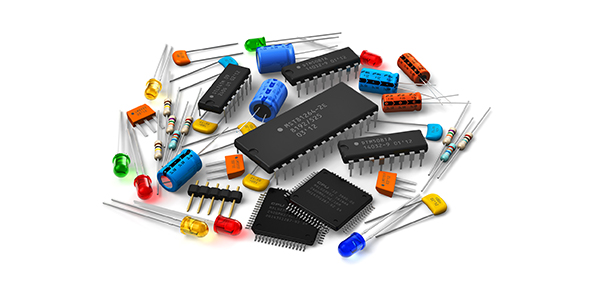# Basic Of Digital Electronic

9 Questions | Total Attempts: 65SettingsBasic concept of DE

• 1.
............ are Universal gates.
• A.

NAND & NOR

• B.

EX-OR

• C.

NOT gate

• D.

None of above

• 2.
When the output of NAND gate is LOW
• A.

When both input are same

• B.

When one is high and other is low

• C.

When both input are 1

• D.

When both input are 0

• 3.
What will the next number of this series, when the base is octal 656, 657, .....
• A.

658

• B.

670

• C.

660

• D.

700

• 4.
Which Universal gate is sufficient to implement, when function is in POS form
• A.

OR gate

• B.

NAND gate

• C.

EX-NOR gate

• D.

NOR gate

• 5.
A parity bit is an extra bit that is attached with a message to make total number of......... either even or odd.
• A.

0

• B.

1

• C.

Both 0 & 1

• D.

None of above

• 6.
Which one is non-weighted code
• A.

84-2-1

• B.

BCD

• C.

Excess-3

• D.

2421

• 7.
To design a BCD adder which number would you add if the sum is incorrect
• A.

0110(6)

• B.

0101(5)

• C.

1000(8)

• D.

1001(9)

• 8.
This device always gives single output.
• A.

Encoder

• B.

Multiplexer

• C.

De-Multiplexer

• D.

Code converter

• 9.
A 1024x6 ROM has....... input
• A.

6

• B.

1024

• C.

5

• D.

10

Related TopicsBack to top